# Probability

Background reading: Galavotti, "Probability" and Gillies, "Varieties of Propensity"

# Warm up puzzle

Two people each flip a coin 50 times. One of them (the honest one) faithfully writes down the result of each coin toss in order. The other one (the liar) just makes up a sequence of 50 results and writes them down, hoping to trick us into thinking the coin had actually been flipped. Their results are displayed below.

Question: Which sequence belongs to the honest one, and which sequence belongs to the liar?

 Sequence A hTThTThhhT TThhTTThTT hTTTThhhhh ThTThThTTT hThhTTThhh Sequence B hTThhhTThT ThThhThTTT hhThhllThT ThhThTTThh ThhTTThThT

Before scrolling further, make a guess, and think about why you think one is more likely to be authentic than the other one.

It turns out that you can quickly determine the answer from the following fact: there is an 83% chance of getting 4 heads in a row, and a similar chance of getting 4 tails in a row, somewhere in a sequence of 50 coin tosses. Whereas most humans who try to "simulate" randomness attempt to distribute heads and tails as evenly as possible, it turns out that the more tosses you make, the more likely it is to get long runs of many heads or tails in a row. (So, the solution is: Sequence A is most likely to correspond to the honest one and Sequence B to the liar.)

Don't believe me? You can try it yourself using this simulation of a coin flip. Record your results. At what point did you find 5 heads in a row? Or 5 tails in a row?

The theory that captures these kinds of non-intuitive facts about uncertain outcomes is probability theory. Today we're going to talk about what probability theory is, with an aim toward trying to understand why it works so well.

# A scoundrel's mathematics

The history of mathematics is not an honourable one, having been developed closely with the history of codes and with weapons of war. But the real scoundral's mathematics was always the study of probability, which was largely developed to win at games of chance and bet on people dying. Although this kind of gambling had been played for thousands of years, it became institutionalised in Europe with the advent of casinos. And it coincided with the new industry of maritime life insurance that arose in the 16th and 17th centuries.

One of the most famous such stories concerns a French aristocrat named Antoine Gombaud, who liked to gamble on games involving dice throws. Unfortunately, the legend goes, Gombaud was horrible at these games and kept losing money, in spite of the fact that he had followed all the local rules of thumb concerning how to win.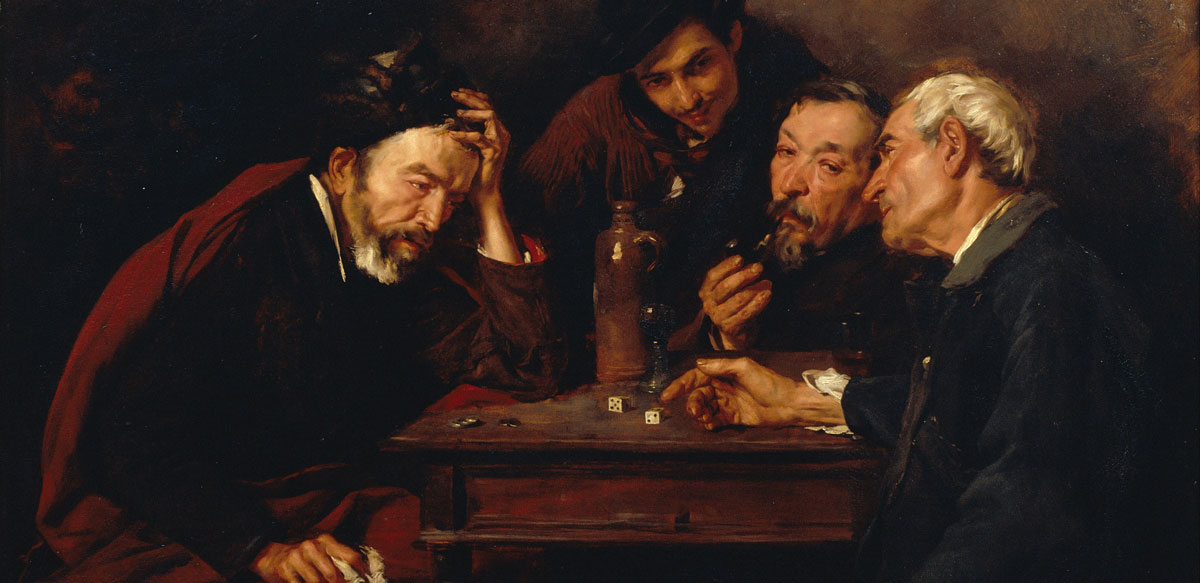So, Gombaud asked two of the greatest mathematicians of the time to have a look at the problem, Blaise Pascal and Pierre de Fermat. They quickly figured out the problem.

Gombaud was betting on the assumption that if you throw a pair of dice 24 times, you'll more likely than not to get two sixes on one of the throws. That assumption was wrong. Pascal and Fermat showed how to analyse the problem.

When you throw a pair of fair dice, there are 36 equally likely outcome, one of which is double-sixes.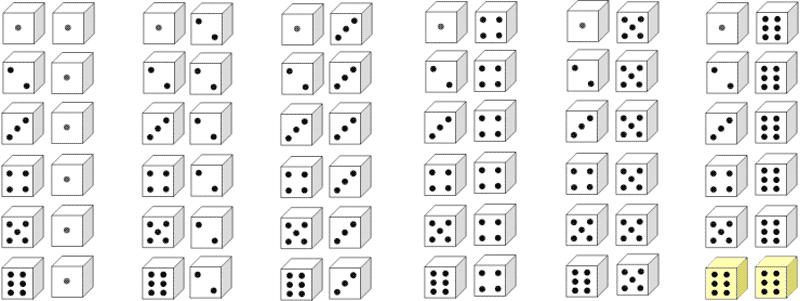So, the odds that you won't throw double-sixes in a given throw is 35/36. And, for reasons that we will see soon, the odds of not throwing double sixes on 24 independent throws is given by multiplying this value 24 times:

$\frac{35}{36} \times \frac{35}{36}\times \cdots (24 \text{ times total}) \cdots \times \frac{35}{36} = \left(\frac{35}{36}\right)^{24} \approx 0.51.$

This means that there is a 51% chance that you won't throw double-sixes in 24 runs, which explains why Gombaud was losing money — he had unwittingly set the game up so that the odds were against him!

Whether or not you've done calculations involving probabilities like these before, you can see that there are many similar circumstances in which probabilities are crucial for everyday from gambling to insurance to your likelihood of catching the bus in the morning.Probability has now become one of our most reliable frameworks for dealing with questions of uncertainty. It's incredible effectiveness can be especially vivid when there is a lot of money on the line. But it also has crucial applications in philosophy of science, such as in the study of confirmation, scientific explanation, and causation. So, it is worth spending a little time to remind yourself what probability theory is like.

However, our task for now will be to understand something more fundamental: what exactly does probability describe? It's just a mathematical theory, after all, which happens to work well for lots of known phenomena dice and card games. But what properties do all probabilistic phenomena share? This is the question of the interpretation of probability, and it will be our main concern today.

# Overview of probability theory

Probability theory is a lot like Euclidean geometry, in that it is a defined as an axiomatic mathematical framework, which turns out to be incredibly accurate at describing the real world. Let's first have a look at the basic axioms of probability, and then see some of the most important consequences.

All of probability theory comes out of just three axioms, first stated in this way by the Russian mathematician Andrey Kolmogorov in 1933.

1. Functions from events to [0,1]. Probabilities are firstly an assignment of a number (the "probability") to an appropriate event or state of the world. People sometimes speak about probabilities as lying on a 0 to 100 scale. We will from now on adopt the convention on which probabilities take values between 0 and 1. So, if $$x$$ is an event like "rolling double sixes," the probability is a function that assigns it a number $$n$$ within the interval [0,1]:

$\Pr(x) = n.$

For example, the probability of flipping a fair coin heads is given by $$\Pr(heads)=1/2$$. And in the game of poker, if each player is dealt 5 cards, each hand containing at least one pair turns out to have probability given by:

$\Pr(pair)=0.42.$A practical consequence is that there's a pretty good chance of getting a pair in any given hand. So if you're in a large group, you can expect at least one of your opponents to have a pair.

2. Something must happen. Even in situations of uncertainty, at least one of the possible outcomes must happen. For example, a regular die will always roll 1, 2, 3, 4, 5, or 6. This second axiom is often expressed as the condition that if $$S$$ is the set of all possible outcomes, then,

$\Pr(S)=1.$

3. Exclusive disjunctions add. If two events cannot both happen at once, like rolling a 1 and rolling a 2 on a regular die, then the probability of their disjunction (i.e. of event 1 or event 2) is just the sum of their individual probabilities. That is, if $$Pr(x \text{ & } y)=0$$ (i.e. two events can't both happen), then,

$\Pr(x \text{ or } y) = \Pr(x) + \Pr(y).$

And we assume the same holds for any number of repeated disjunctions. So, for example, we can ask what's the probability of rolling either 1 or 6 on a fair die? These two outcomes are mutually exclusive, so it's given by,

$\Pr(1 \text{ or } 6) = \Pr(1) + \Pr(6) = \frac{1}{6}+\frac{1}{6}=\frac{2}{6} = \frac{1}{3}.$

In summary, the mathematical theory of probability is defined by the three axioms,

1. $$Pr(x)=n$$ is in the interval [0,1]
2. $$Pr(S)=1$$ for the space of all possibilities $$S$$
3. $$Pr(x \text{ or } y) = Pr(x)+Pr(y)$$ for all mutually exclusive possibilities.

That's it! The entire theory of probability follows from just those three ideas. Of course, it takes a little bit of manipulation to see that. Let's just look at a few of the essential parts.

# Some interesting consequences

### The law of large numbers

The law of large numbers probabilities represent the facts about large numbers of trials.

For example, a crucial fact about shooting a pair of dice is that they will sum to 7 more often than any other number. It's easy to see why in the chart below: there are simply more combinations that sum to 7 than any other number! According to probability theory, the numbers on the dice will sum to 7 with probability $$Pr(7)=1/6$$.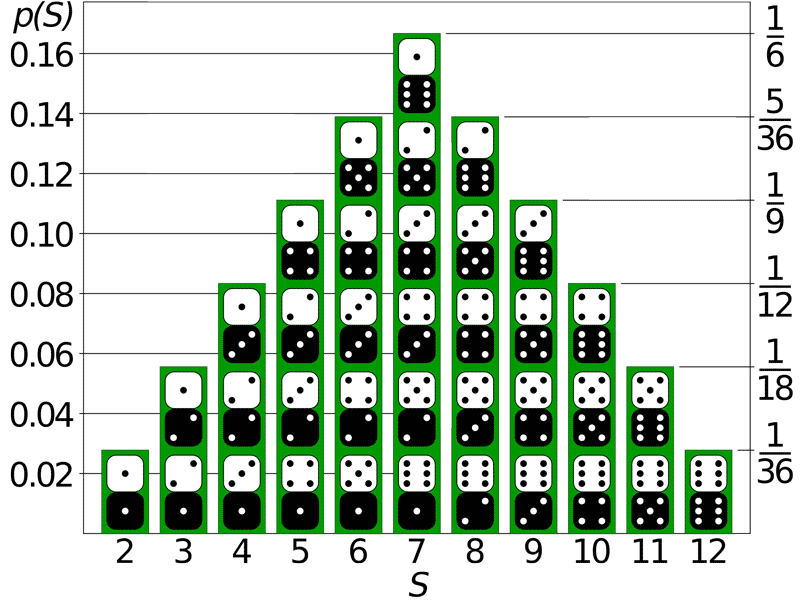A consequence of the law of large numbers is thus that if you were to average your rolls over a very large number of throws, it would approach 7. In other words, the average sum of a pair of dice tends to 7 after a large number of trials.

The law of large numbers is what allows casinos use to make money. Casino the games are typically set up so that the casino might lose on any given trial, but in the limit of a large number of trials the casino will make money.

### Conditional probability and the Monty Hall Problem

One of the most common ways to use probability is in determining a likelihood given some evidence. This is described using what is known as conditional probability. To write "The probability of $$A$$ given $$B$$" we will write $$\Pr(A|B)$$. The official definition of conditional probability is,

$\Pr(A|B) = \frac{\Pr(A \text{ and } B)}{\Pr(B)}.$

This is just a definition, but it turns out to provide a very good definition of the intuitive meaning of conditional probability.

For example, we know from the chart above that the probability of rolling double-sixes on a given turn is only 1/36. But suppose we get more information, such as by rolling one of the die first and getting a 6. What then is the probability of rolling double-sixes?Call each die $$B$$ and $$A$$ respectively. Using the definition above, we find that it is given by,

$\Pr(A=6 \;|\; B=6)=\frac{\Pr(A=6 \text{ and } B=6)}{\Pr(B=6)} = \frac{1/36}{1/6} = \frac{1}{6}.$

This result makes sense. After all, we already know that when there's only one die to throw, the probability of any given number is 1/6. However, the change from 1/36 to 1/6 reflects a common occurrence in probability theory, that introducing new information can often change the probabilities from what they were before that information was introduced.

Perhaps the most famous and notorious example of this comes from a problem in probability known as the Monty Hall Problem. It is named after the host Monty Hall of the show, "Let's Make a Deal." Here's what it was like.

The Monty Hall Problem in probability theory asks the following simple setup. Suppose that you're offered the choice of three closed doors. You know that behind one of them is a car (which is most desirable), and that behind the other two is a goat.

You make a choice. But, before revealing your choice, Monty reveals a goat behind one of the other two doors (and this is important — Monty is guaranteed to reveal a goat at this step). He then offers you a choice: do you stay with your original choice, or switch to the other door?Most people think that it doesn't matters. Since there are two doors, they assume the car is equally likely to be behind either one. However, when you do the probabilistic calculation, you find that on the contrary, the best decision is to switch doors, giving you a 2/3 probability of winning the car, as opposed to a 1/3 probability of winning if you stay.

This surprising result is a simple fact of probability that you can just calculate. (Consider giving it a try!) You can also convince yourself by just repeating a number of trials, for example using this Monty Hall Problem game. The game was made available by the New York times, as part of an article revealing how even scientists can become confused by the non-intuitive nature of probability theory.

### Probabilistic independence and the Gambler's Fallacy

A final feature of probability theory worth mentioning is the concept of independence. Certain probabilistic events are independent in the sense that they have nothing to do with each other. We can write this in terms of conditional probabilities as the property that,

$\Pr(A|B)=\Pr(A).$

In other words, A and B are independent if the probability of A given B doesn't tell you anything new — it's just the same as the probability of A. This property is called probabilistic independence, or sometimes "stochastic independence." Using the definition of conditional probability above, it is easy to show that it is equivalent to the statement,

$\Pr(A \text{ and } B) = \Pr(A)\times\Pr(B).$

This is the rule that was used in Pascal and Fermat's solution to the Gombaud problem. They recognised that individual dice throws are independent of each other. So, if the probability of not throwing double-sixes is 35/36, the probability of not throwing double-sixes on two different throws is the product,

$\frac{35}{36}\times\frac{35}{36} \approx 0.95.$

And the probability of not throwing double-sixes anywhere in 24 throws is just the same product 24 times over.

The phenomenon of independence is another non-intuitive fact of probability. For example, it is embodied in the following question: If I flip heads 99 times in a row with a fair coin, what is the probability that I will flip heads on the 100th time? Most people have a strong intuition that it should be a very small probability. This is a fallacy. The correct answer is probability 1/2, the same as any other coin flip. The outcome of 99 previous flips has no effect whatsoever on the outcome of the next coin flip.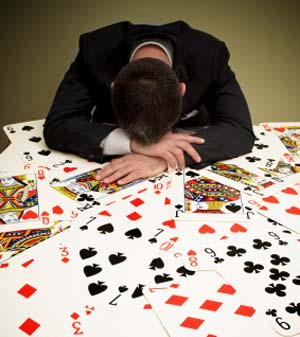To assume otherwise is known as the gambler's fallacy. It's what leads gamblers to tell themselves that after a long losing streak, they're likely to come up winning soon. That is simply wrong. If the gambling events are independent, then the losing streak has no effect whatsoever on their future. At best, it suggests (by induction) that the gambler has poor gambling skills, and may continue to lose in the future!

# Interpretations of probability

What is it about systems like coins, cards and insurance claims that makes probability applicable to them? What makes a phenomenon probabilistic? This is the question of how to interpret probability. There are several approaches that have been proposed. Here are a few of the major ones.

### Classical interpretation

The classical interpretation of probability was stated clearly by Pierre-Simon Laplace, one of the fathers of probability theory, in 1814."The theory of chance consists in reducing all the events of the same kind to a certain number of cases equally possible, that is to say, to such as we may be equally undecided about in regard to their existence, and in determining the number of cases favorable to the event whose probability is sought. The ratio of this number to that of all the cases possible is the measure of this probability, which is thus simply a fraction whose numerator is the number of favorable cases and whose denominator is the number of all the cases possible."

The slogan, in short, is that probabilistic phenomena are defined by sets of equally possible events. For example, when flipping a fair coin, Laplace says that an outcome of heads and tails are events of the same kind that are equally possible. For this reason, they behave according to the laws of probability theory.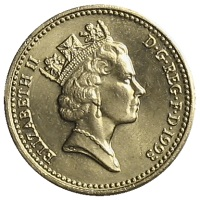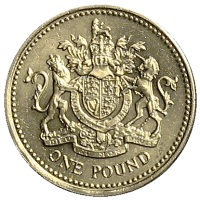A difficulty with this interpretation (which you might wish to try your hand at repairing!) is that the meanings of the terms in this slogan are not well-defined. What exactly does it mean to be "of the same kind"? Or "equally possible"?

One concern about the latter is that on the ordinary way of thinking about it, possibility is not a matter of degree. That is, something is either possible or it is not; it does not make sense to say that one thing is "more possible" or "equally possible" as compared to another. So, the classical interpretation does not seem to reflect any ordinary notion of possibility.

On the other hand, if "possibility" just means "probability" then we are begging the question, since the whole aim of an interpretation is to determine what it means for something to be probable.

One possible way of dealing with this is to allow that possibilities have unequal weights, which represent primitive facts about the world. Probability theory can then be interpreted as the logic describing the interplay of weighted possibilities. This is known as the logical interpretation of probability, often considered to be an example (or else a slight modification) of a classical interpretation.

### Subjective Interpretation

The classical interpretation of probability gave it metaphysical significance corresponding to particular kinds of facts about the world. An alternative is to consider probability to describe an epistemic condition, corresponding to certain kinds of justified belief that an agent may have.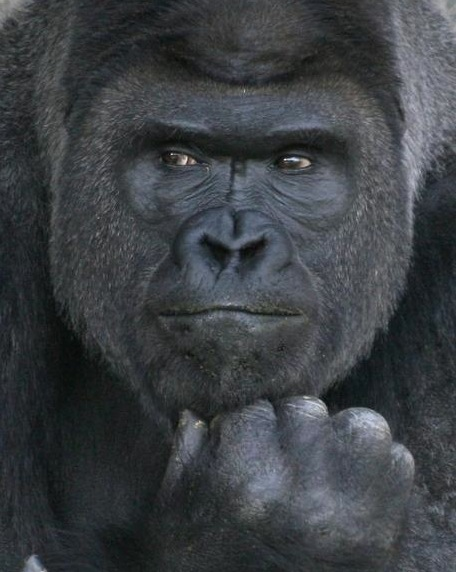These interpretations begin by taking probabilities to represent the degree of belief that a rational agent should have about a situation. For example, given the roll of a regular pair of dice, a rational agent should assign a degree of belief (or "credence") of 1/36 that it will come up double-sixes. And, when the first die comes up a six, that belief should be updated using conditional probability to a credence of 1/6 that the roll will be double-sixes.

When an agent's belief is about the outcome of a gamble in which money is being bet, then there are lots of arguments that show that you will lose money unless your beliefs track the laws of probability. This provides an argument (known as a Dutch book argument) that a rational agent's degree of belief will be probabilistic, since no rational agent would adopt a losing strategy at gambling.

There are various problems with treating probabilities as degrees of belief. One of the biggest ones is the problem of ignorance. Suppose you don't know whether or not a coin is fair: it might be biased toward heads, or biased toward tails, and you have absolutely no knowledge one way or the other. Then there is no number between 0 and 1 that you can assign to your degree of belief that the outcome would be heads.

Try it. You could try to assign probability 1/2 to heads. But that's the same thing you would do if you knew that it had probability 1/2 of coming up heads, which is not your current state of belief. And none of the other numbers between 0 and 1 seem any better. So, the degree of belief that an agent may have appears to allow for ignorance in a way that probability does not.

Another problem is that many probabilities seem to have nothing to do with our belief. For example, in quantum mechanics there are objective probabilities that get assigned to certain particles, like whether or not it spins clockwise or anticlockwise. A rational agent may believe whatever he or she wants about such particles. But the spin-clockwise outcome still has an independent objective chance of occurring, which does not seem to be captured by an agent's degree of belief.

### Frequency Interpretation

A well-known property of probability is that it is closely related to how often certain events happen in the world. Frequency interpretations take advantage of this. A typical expression of a frequency interpretation is that:

Frequency Interpretation. The probability of X with respect to a reference class Y is the relative frequency of actual occurrences of X in Y.

So, for example, the probability of a coin flipping heads in 100 trials is the actual number of occurrences of heads in those 100 trials. Notice that one distinction between the frequency interpretation and the classical interpretation is that the former requires actual occurrences, while the latter only requires possible occurrences.

The technical difficulties with the frequency interpretation arise from the fact that does not satisfy Axioms 2-3 of the probability axioms. At best, relative frequencies only approximate what is described by probability theory.

These difficulties manifest in several ways. For example, take a coin that is identical in every way to a fair coin, but which is hooked up to a bomb that destroys it after one flip. Suppose that you flip it and that it lands heads, before being blown into oblivion. What was the probability that it would land heads?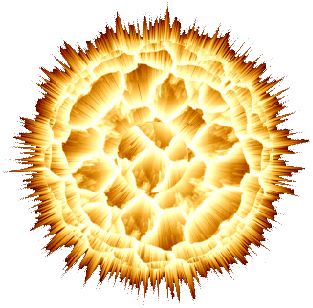According to the frequency interpretation, it would seem to be probability 1. The reference class consists of just event, and that event came up heads. But that seems non-intuitive, since it seems equally likely that the coin might have landed tails.

A further non-technical problem is that the frequency interpretation requires specifying a reference class. But how do we choose that? It seems to be arbitrary, or at least is not given in the statement of the interpretation itself.

### Propensity Interpretation

The final class of interpretations that we will discuss are the propensity interpretations. The idea is to think of probability as a "habit" or "tendency" for something to occur, which is often primitive and cannot be further analysed. Karl Popper, a well-known advocate of a propensity interpretation, put it as follows in his 1954 Logic of Discovery.

"[W]e we have to visualise the conditions as endowed with a tendency or disposition, or propensity, to produce sequences whose frequencies are equal to the probabilities; which is precisely what the propensity interpretation asserts."

On this view, the exploding coin in the previous example still had a propensity (and therefore a probability) to land heads, even though we were not able to actually measure that propensity.

Many of the details of the propensity theory hinge on saying just what propensities are. In some cases a tendency may be explained psychologically; in other cases by a physical theory; or by any number of other things. Without knowing what exactly the concept of a propensity is, it is hard to know whether or not it will track probabilities. And of course, we cannot just use it as a synonym for "probability" since to do that would again beg the question of what probabilities are.A further well-known difficulty is that propensities are often thought to be asymmetric in time, whereas probabilities are not. For example, a dose of cyanide has a propensity to kill you, and that propensity can be represented as a probability.

However, your death does not appear to have a propensity for cyanide consumption, even though we may say that a dead person had previously drunk cyanide with some probability.

## What you should know

• The origin of probability and some of its central claims
• The interpretations of probability and their difficulties